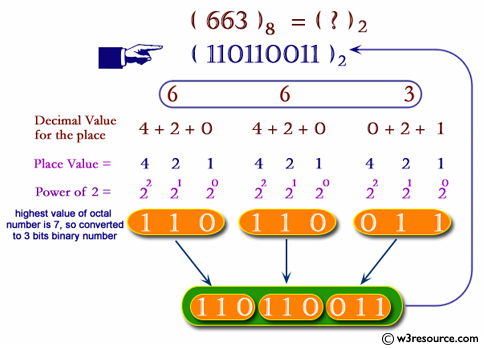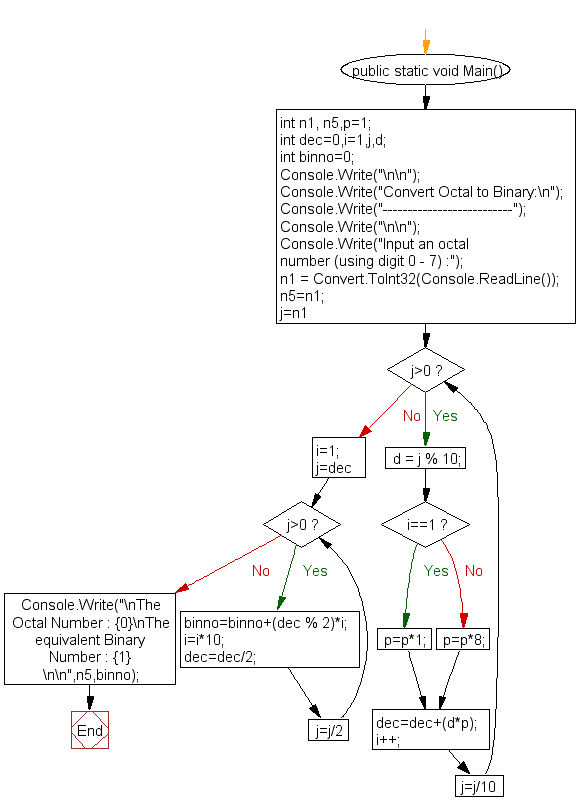﻿ C# - Convert an octal number into binary - w3resource# C# Sharp Exercises: Convert an octal number into binary

## C# Sharp For Loop: Exercise-54 with Solution

Write a program in C# Sharp to convert an octal number into binary.Sample Solution:-

C# Sharp Code:

``````using System;
public class Exercise54
{
public static void Main()
{

int n1, n5,p=1;
int dec=0,i=1,j,d;
int binno=0;

Console.Write("\n\n");
Console.Write("Convert Octal to Binary:\n");
Console.Write("--------------------------");
Console.Write("\n\n");

Console.Write("Input an octal number (using digit 0 - 7) :");
n5=n1;

for (j=n1;j>0;j=j/10)
{
d = j % 10;
if(i==1)
p=p*1;
else
p=p*8;

dec=dec+(d*p);
i++;
}

/*------------------------------------------------------------------------------*/
i=1;

for(j=dec;j>0;j=j/2)
{
binno=binno+(dec % 2)*i;
i=i*10;
dec=dec/2;
}

Console.Write("\nThe Octal Number : {0}\nThe equivalent Binary  Number : {1} \n\n",n5,binno);
}
}
```
```

Sample Output:

```Convert Octal to Binary:
--------------------------
Input an octal number (using digit 0 - 7) :45
The Octal Number : 45
The equivalent Binary  Number : 100101
```

Flowchart:C# Sharp Code Editor:

Contribute your code and comments through Disqus.

What is the difficulty level of this exercise?

Test your Programming skills with w3resource's quiz.

﻿